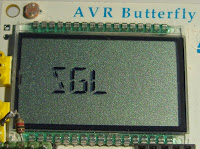## 18 January, 2014Photo @LA3ZA
Here's an adapter that emulates both a single-lever paddle mode and the ultimatic mode. It is meant to go between a dual-lever paddle and an iambic keyer. The adapter has been implemented in an AVR Butterfly in C and it is compatible with Morse keyers such as the one in the Elecraft K3 and the K1EL WKUSB. The single-lever emulation is probably the most novel part and it is meant to make it easier to practice single-lever keying on a dual-lever paddle.

When pressing both paddles in the ultimatic mode the last one to be pressed takes control, rather than the alternating dit-dah or dah-dit of the iambic mode. The five possible states are then (from "An ultimatic adapter for iambic keyers", Kevin E. Schmidt, W9CF, 2008):
1. Lin = 1, Rin = 1 => Lout = 0, Rout = 0.
2. Lin = 1, Rin = 0 => Lout = 0, Rout = 1.
3. Lin = 0, Rin = 1 => Lout = 1, Rout = 0.
4. Lin = 0, Rin = 0 after Lin = 1, Rin = 0 => Lout = 1, Rout = 0.
5. Lin = 0, Rin = 0 after Lin = 0, Rin = 1 => Lout = 0, Rout = 1.
The left and right inputs are Lin and Rin and an open input is "1" and a closed one is "0" since the key grounds the input. The output is "1" when it is on and "0" when it is off. The adapter makes it possible with this mode on your favorite keyer where you may have missed this mode.

When I implemented the ultimatic adapter after W9CF's instructions, it struck me that it would be both useful and easy to add an emulator for a single-lever paddle also. When both keys are pressed in this mode, the last one to be pressed is ignored. This gives the following inverted outputs in states 4 and 5:
1. Lin = 0, Rin = 0 after Lin = 1, Rin = 0 => Lout = 0, Rout = 1.
2. Lin = 0, Rin = 0 after Lin = 0, Rin = 1 => Lout = 1, Rout = 0.
The code also has a direct mode that just sends the input unchanged to the output, as well as a possibility for exchanging the right and left paddles. The display may therefore show 'ULT, 'SGL, 'DIR' and 'ULTx', 'SGLx', 'DIRx' for these combinations.

The exchange mode is actually quite fun to use. In general for me it is easier to swap the paddles when keying with my left hand. I don't think I am the only one with that experience.

The C code can be found below. These days I should probably have written it for the Arduino, but the code should be easy to move. Perhaps I'll do that myself, now that I have an Arduino Mega on order.

Many keyers have the ultimatic mode and the possibility to exchange right and left, but no keyers have the single-paddle emulation mode as far as I know. I think it is quite useful. This summer when I implemented it I thought it was novel also.

But that was before I found out that this mode actually had been proposed by Larry Winslow, W0NFU, in QST in October 2009 and that one can get an iambic to single paddle kit from WB9KZY. Oh well, "there is nothing new under the sun" as the wise man of Ecclesiastes said some 3000 years ago. Just like the ultimatic mode has been implemented in many keyers these days, let me propose the single paddle mode for implementation as a new command also.

The C code is here (formatted with Hilite Me):

 ```void paddle() { if (keyer == 0) // Direct: output = input { l_out = !(0x01 & l_in); r_out= !(0x01 & r_in); // Boolean inverse } else { /* Direct implementation of table 3 in "K Schmidt (W9CF) "An ultimatic adapter for iambic keyers" http://fermi.la.asu.edu/w9cf/articles/ultimatic/ultimatic.html with the addition of the Single-paddle emulation mode */ if (state==0) { if ((l_in==0) & (r_in==0)) // two paddles closed, right first { state = 0; if (keyer==1) // Ultimatic { l_out = 1; r_out = 0; // change to left } else if (keyer==2) // Single-paddle emulation { l_out = 0; r_out = 1; // keep right } } else if ((l_in==0) & (r_in==1)) { state = 1; l_out = 1; r_out = 0; } else if ((l_in==1) & (r_in==0)) { state = 0; l_out = 0; r_out = 1; } else if ((l_in==1) & (r_in==1)) { state = 0; l_out = 0; r_out = 0; } } else if (state==1) { if ((l_in==0) & (r_in==0)) // two paddles closed, left first { state = 1; if (keyer==1) // Ultimatic { l_out = 0; r_out = 1; // change to right } else if (keyer==2) // Single-paddle emulation { l_out = 1; r_out = 0; // keep left } } else if ((l_in==0) & (r_in==1)) { state = 1; l_out = 1; r_out = 0; } else if ((l_in==1) & (r_in==0)) { state = 0; l_out = 0; r_out = 1; } else if ((l_in==1) & (r_in==1)) { state = 0; l_out = 0; r_out = 0; } } } } ```

The code is also on Github.